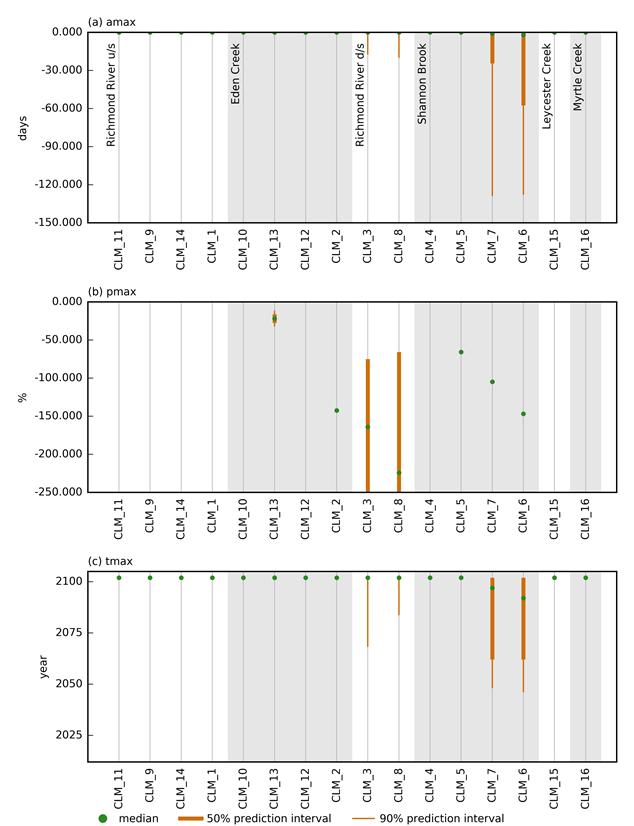# 2.6.1.6.9 Zero-flow days (ZFD)

Page 34 of 41

The median absolute change in Figure 18 indicates that the number of ZFD across the region will not increase, with the exception of nodes CLM_007 and CLM_006 at the downstream end of Shannon Brook where the median change in the number of ZFD is 3 days. The 95th percentile of change in ZFD is 120 days. As noted earlier, small changes in simulated flow can result in large changes in the number of ZFD, as ZFD are defined as days with streamflow less than 0.01 ML/day.Figure 18 Predictive distribution of (a) maximum raw change (amax), (b) maximum percent change (pmax) and (c) year of maximum change (tmax) for the number of zero-flow days (ZFD)

d/s = downstream of; u/s = upstream of

The circle indicates the median of the posterior predictive distribution, the length of the thick vertical line spans the interquartile range (or 50th percentile prediction interval), and the thin vertical line spans the 90th percentile prediction interval. Nodes are grouped per catchment, ordered from upstream to downstream.

Data: Bioregional Assessment Programme (Dataset 2)

Last updated:
18 October 2018